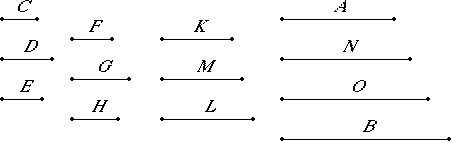# Proposition 19

Between two similar solid numbers there fall two mean proportional numbers, and the solid number has to the solid number the ratio triplicate of that which the corresponding side has to the corresponding side.

Let A and B be two similar solid numbers, and let C, D, and E be the sides of A, and let F, G, and H be the sides of B.

VII.Def.21

Now, since similar solid numbers are those which have their sides proportional, therefore C is to D as F is to G, and D is to E as G is to H.

I say that between A and B there fall two mean proportional numbers, and A has to B the ratio triplicate of that which C has to F, D has to G, and E has to H.

Multiply C by D to make K, and multiply F by G to make L.Now, since C and D are in the same ratio with F and G, and K is the product of C and D, and L the product of F and G, K and L are similar plane numbers, therefore between K and L there is one mean proportional number M.

VIII.18

Therefore M is the product of D and F was proved in the theorem preceding.

VII.17

Now, since D multiplied by C makes K, and multiplied by F makes M, therefore C is to F as K is to M. But K is to M as M is to L. Therefore K, M, and L are continuously proportional in the ratio of C to F.

VII.13

And since C is to D as F is to G, alternately therefore C is to F as D is to G. For the same reason also D is to G as E is to H.

Therefore K, M, and L are continuously proportional in the ratio of C to F, in the ratio of D to G, and also in the ratio of E to H.

Next, multiply E and H by M to make N and O respectively.

Now, since A is a solid number, and C, D, and E are its sides, therefore E multiplied by the product of C and D makes A. But the product of C and D is K, therefore E multiplied by K makes A. For the same reason also H multiplied by L makes B.

VII.17

Now, since E multiplied by K makes A, and further also multiplied by M makes N, therefore K is to M as A is to N.

But K is to M as C is to F, as D is to G, and as E is to H, therefore C is to F as D is to G, as E is to H, and as A is to N.

VII.18

Again, since E and H multiplied by M make N and O respectively, therefore E is to H as N is to O.

But E is to H as C is to F and as D is to G, therefore C is to F as D is to G, as E is to H, as A is to N, and as N is to O.

VII.17

Again, since H multiplied by M makes O, and further also multiplied by L makes B, therefore M is to L as O is to B. But M is to L as C is to F, a D is to G, and as E is to H. Therefore C is to F as D is to G, and as E is to H, as are O to B, A to N, and N to O.

Therefore A, N, O, and B are continuously proportional in the aforesaid ratios of the sides.

I say that A also has to B the ratio triplicate of that which the corresponding side has to the corresponding side, that is, of the ratio which the number C has to F, or D has to G, and also E has to H.

V.Def.10

Since A, N, O, and B are four numbers in continued proportion, therefore A has to B the ratio triplicate of that which A has to N. But it was proved that A is to N as C is to F, as D is to G, and as E is to H.

Therefore A also has to B the ratio triplicate of that which the corresponding side has to the corresponding side, that is, of the ratio which the number C has to F, D has to G, and also E has to H.

Therefore, between two similar solid numbers there fall two mean proportional numbers, and the solid number has to the solid number the ratio triplicate of that which the corresponding side has to the corresponding side.

Q.E.D.

## Guide

Assume cde and fgh are similar solid numbers so that c : d : e = f : g : h, or, expressed alternately,

c : f = d : g = e : h.
Then the numbers
cde, fde, fge, and fgh

are in continued proportion giving two mean proportionals between cde and fgh. Also, the ratio cde : fgh is compounded of the three equal ratios of the sides c : f, d : g, and e : h, so it is a triplicate ratio of each.

#### Use of this proposition

This proposition is used in a few propositions in Books VIII and IX starting with VIII.25. Proposition VIII.21 is a partial converse of this one.